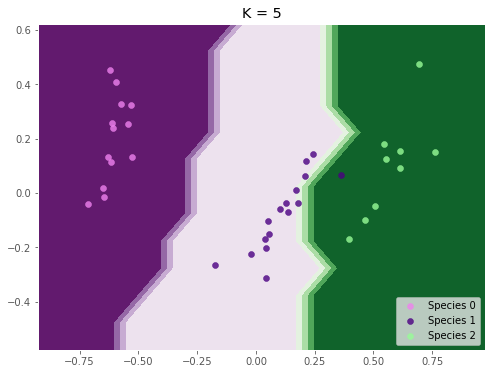## K-nearest Neighbours Classification in python

• Post author:
• Post category:Python

# K-nearest Neighbours Classification in python

K-nearest Neighbours is a classification algorithm.

Just like K-means, it uses Euclidean distance to assign samples, but K-nearest neighbours is a supervised algorithm and requires training labels.

K-nearest neighbours will assign a class to a value depending on its k nearest training data points in Euclidean space, where k is some number chosen by the user.

We will be using the iris data set to try and predict species of iris from petal and sepal dimensions. So, first thing’s first, let’s load the data.

In :
from sklearn import datasets
import pandas as pd

X = pd.DataFrame(iris['data'], columns=iris['feature_names'])
# y is the target species from 0, 1 or 2
y = iris['target']

Out:
sepal length (cm) sepal width (cm) petal length (cm) petal width (cm)
0 5.1 3.5 1.4 0.2
1 4.9 3.0 1.4 0.2
2 4.7 3.2 1.3 0.2
3 4.6 3.1 1.5 0.2
4 5.0 3.6 1.4 0.2

Now because the petal width/length and the sepal width/lengths are different scales (the sepals of petals tend to be much larger than the petals), we need to pre-process the data using a preprocessor.

We will be using the MinMaxScaler, which scales the features according to:

$z_i = \dfrac{x_i – min(x)}{max(x) – min(x)}$

In :
from sklearn import preprocessing

scaler = preprocessing.MinMaxScaler()
X = scaler.fit_transform(X)


Now we should split our data into a training set and a test set in order to properly assess our model

In :
from sklearn.model_selection import train_test_split

X_train, X_test, y_train, y_test = train_test_split(X, y, random_state=1)


So that we can work with our data in 2-dimensional space, we will reduce the dimensionality of the data down to 2.

We will use principle component analysis, for more information on this, see the related post here.

In :
from sklearn.decomposition import PCA

pca_model = PCA(n_components=2)
pca_model.fit(X_train)
X_train = pca_model.transform(X_train)
X_test = pca_model.transform(X_test)

# 2-Dimensions
X_train[:5]

Out:
array([[ 0.25435516, -0.02893008],
[ 0.49953791, -0.14022187],
[ 0.53635187,  0.06871783],
[-0.60650252,  0.1420104 ],
[ 0.26606906, -0.31639692]])

We now run our k-nearest neighbours analysis using scikit-learn’s KNeighborsClassifier.

In :
from sklearn.neighbors import KNeighborsClassifier

k = 5

knn_model = KNeighborsClassifier(n_neighbors=k)
knn_model.fit(X_train, y_train)

Out:
KNeighborsClassifier(algorithm='auto', leaf_size=30, metric='minkowski',
metric_params=None, n_jobs=1, n_neighbors=5, p=2,
weights='uniform')

We can now use our model to make predictions based on our test data:

In :
y_predict = knn_model.predict(X_test)
y_predict

Out:
array([0, 1, 1, 0, 2, 1, 2, 0, 0, 2, 1, 0, 2, 1, 1, 0, 1, 1, 0, 0, 1, 1, 2,
0, 2, 1, 0, 0, 1, 2, 1, 2, 1, 2, 2, 0, 1, 0])

And becuase we used principle component analysis to reduce the dimensions, we can use our model to plot the decision boundaries based on our training data and where our test data falls relative to these boundaries:

In :
import matplotlib.pyplot as plt
import matplotlib
import numpy as np
%matplotlib inline
matplotlib.style.use('ggplot')

fig = plt.figure(figsize=(8, 6))
resolution = 0.1

colors = {0: 'violet', 1: 'indigo', 2: 'palegreen'}
x_min, x_max = X_train[:, 0].min(), X_train[:, 0].max()
y_min, y_max = X_train[:, 1].min(), X_train[:, 1].max()
x_range = x_max - x_min
y_range = y_max - y_min

# Get decision boundaries from model
xx, yy = np.meshgrid(np.arange(x_min, x_max, resolution),
np.arange(y_min, y_max, resolution))

Z = knn_model.predict(np.c_[xx.ravel(), yy.ravel()])
Z = Z.reshape(xx.shape)

# Plot the contour map
plt.contourf(xx, yy, Z, cmap=plt.cm.PRGn)
plt.axis('tight')

# Plot your testing points as wel
for label in np.unique(y_test):
indices = np.where(y_test == label)
plt.scatter(X_test[indices, 0], X_test[indices, 1], c=colors[label], alpha=0.8,
label='Species {}'.format(label))

plt.legend(loc='lower right')
plt.title('K = {}'.format(k))
plt.show()So it looks like our model is very accurate, only misrepresenting very few (1) species.

But of course, we don’t know if another value of k would have performed better. To evaluate our model and compare against other k, we would use the techniques described in Scoring Classifier Models using scikit-learn